After the success of my post Understanding Categorical Cross-Entropy Loss, Binary Cross-Entropy Loss, Softmax Loss, Logistic Loss, Focal Loss and all those confusing names, and after checking that Triplet Loss outperforms Cross-Entropy Loss in my main research topic (Multi-Modal Retrieval) I decided to write a similar post explaining Ranking Losses functions.

Ranking Losses are used in different areas, tasks and neural networks setups (like Siamese Nets or Triplet Nets). That’s why they receive different names such as Contrastive Loss, Margin Loss, Hinge Loss or Triplet Loss.

## Ranking Loss Functions: Metric Learning

Unlike other loss functions, such as Cross-Entropy Loss or Mean Square Error Loss, whose objective is to learn to predict directly a label, a value, or a set or values given an input, the objective of Ranking Losses is to predict relative distances between inputs. This task if often called metric learning.

Ranking Losses functions are very flexible in terms of training data: We just need a similarity score between data points to use them. That score can be binary (similar / dissimilar). As an example, imagine a face verification dataset, where we know which face images belong to the same person (similar), and which not (dissimilar). Using a Ranking Loss function, we can train a CNN to infer if two face images belong to the same person or not.

To use a Ranking Loss function we first extract features from two (or three) input data points and get an embedded representation for each of them. Then, we define a metric function to measure the similarity between those representations, for instance euclidian distance. Finally, we train the feature extractors to produce similar representations for both inputs, in case the inputs are similar, or distant representations for the two inputs, in case they are dissimilar.
We don’t even care about the values of the representations, only about the distances between them. However, this training methodology has demonstrated to produce powerful representations for different tasks.

## Ranking Losses Formulation

Different names are used for Ranking Losses, but their formulation is simple and invariant in most cases. We distinguish two kinds of Ranking Losses for two differents setups: When we use pairs of training data points or triplets of training data points.
Both of them compare distances between representations of training data samples.

If you prefer video format, I made a video out of this post. Also available in Spanish:

### Pairwise Ranking Loss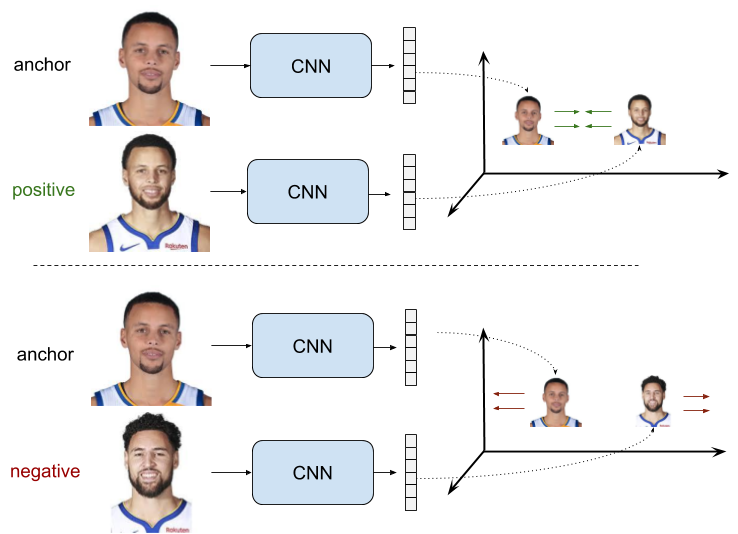Example of a pairwise ranking loss setup to train a net for image face verification. In this setup, the weights of the CNNs are shared. We call it siamese nets. But a pairwise ranking loss can be used in other setups, or with other nets.

Is this setup positive and negative pairs of training data points are used. Positive pairs are composed by an anchor sample $$x_a$$ and a positive sample $$x_p$$, which is similar to $$x_a$$ in the metric we aim to learn, and negative pairs composed by an anchor sample $$x_a$$ and a negative sample $$x_n$$, which is dissimilar to $$x_a$$ in that metric.

The objective is to learn representations with a small distance $$d$$ between them for positive pairs, and greater distance than some margin value $$m$$ for negative pairs. Pairwise Ranking Loss forces representations to have $$0$$ distance for positive pairs, and a distance greater than a margin for negative pairs. Being $$r_a$$, $$r_p$$ and $$r_n$$ the samples representations and $$d$$ a distance function, we can write:

For positive pairs, the loss will be $$0$$ only when the net produces representations for both the two elements in the pair with no distance between them, and the loss (and therefore, the corresponding net parameters update) will increase with that distance.

For negative pairs, the loss will be $$0$$ when the distance between the representations of the two pair elements is greater than the margin $$m$$. But when that distance is not bigger than $$m$$, the loss will be positive, and net parameters will be updated to produce more distant representation for those two elements. The loss value will be at most $$m$$, when the distance between $$r_a$$ and $$r_n$$ is $$0$$. The function of the margin is that, when the representations produced for a negative pair are distant enough, no efforts are wasted on enlarging that distance, so further training can focus on more difficult pairs.

If $$r_0$$ and $$r_1$$ are the pair elements representations, $$y$$ is a binary flag equal to $$0$$ for a negative pair and to $$1$$ for a positive pair and the distance $$d$$ is the euclidian distance, we can equivalently write:

### Triplet Ranking Loss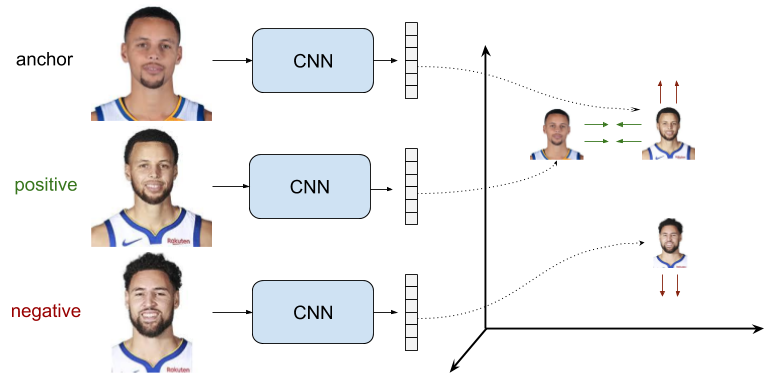Example of a triplet ranking loss setup to train a net for image face verification. In this setup, the weights of the CNNs are shared. We call it triple nets.

This setup outperforms the former by using triplets of training data samples, instead of pairs. The triplets are formed by an anchor sample $$x_a$$, a positive sample $$x_p$$ and a negative sample $$x_n$$. The objective is that the distance between the anchor sample and the negative sample representations $$d(r_a, r_n)$$ is greater (and bigger than a margin $$m$$) than the distance between the anchor and positive representations $$d(r_a, r_p)$$. With the same notation, we can write:

Let’s analyze 3 situations of this loss:

• Easy Triplets: $$d(r_a,r_n) > d(r_a,r_p) + m$$. The negative sample is already sufficiently distant to the anchor sample respect to the positive sample in the embedding space. The loss is $$0$$ and the net parameters are not updated.
• Hard Triplets: $$d(r_a,r_n) < d(r_a,r_p)$$. The negative sample is closer to the anchor than the positive. The loss is positive (and greater than $$m$$).
• Semi-Hard Triplets: $$d(r_a,r_p) < d(r_a,r_n) < d(r_a,r_p) + m$$. The negative sample is more distant to the anchor than the positive, but the distance is not greater than the margin, so the loss is still positive (and smaller than $$m$$).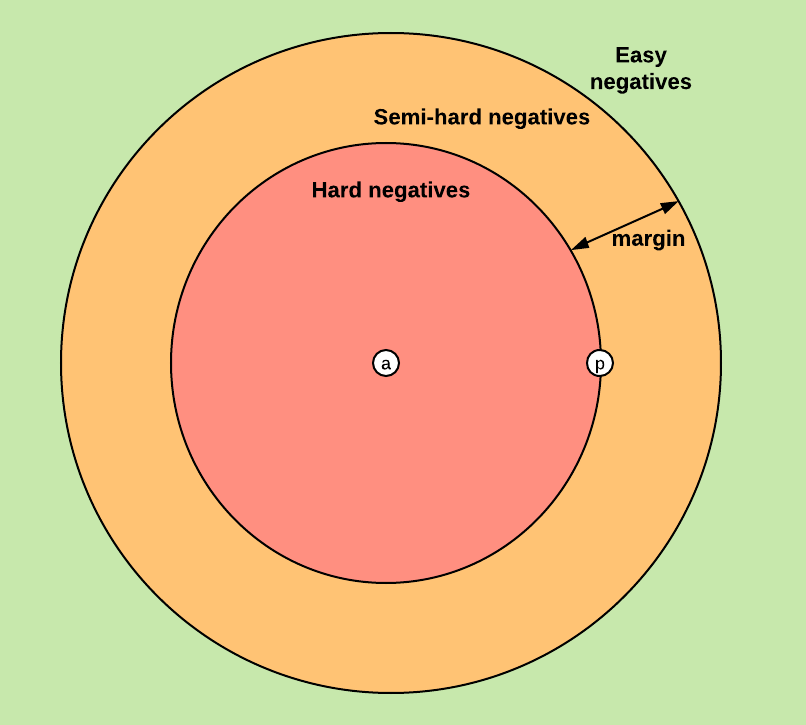Source: https://omoindrot.github.io/triplet-loss. Representation of three “types of negatives” for an anchor and positive pair.

#### Negatives Selection

An important decision of a training with Triplet Ranking Loss is negatives selection or triplet mining. The strategy chosen will have a high impact on the training efficiency and final performance. An obvious appreciation is that training with Easy Triplets should be avoided, since their resulting loss will be $$0$$.

First strategies used offline triplet mining, which means that triplets are defined at the beginning of the training, or at each epoch. Later, online triplet mining, meaning that triplets are defined for every batch during the training, was proposed and resulted in better training efficiency and performance.
The optimal way for negatives selection is highly dependent on the task. But I’m not going to get into it in this post, since its objective is only overview the different names and approaches for Ranking Losses. Refer to Oliver moindrot blog post for a deeper analysis on triplet mining.

Triplet Loss in deep learning was introduced in Learning Fine-grained Image Similarity with Deep Ranking and FaceNet: A Unified Embedding for Face Recognition and Clustering.

This github contains some interesting plots from a model trained on MNIST with Cross-Entropy Loss, Pairwise Ranking Loss and Triplet Ranking Loss, and Pytorch code for those trainings.

## Other names used for Ranking Losses

Ranking Losses are essentialy the ones explained above, and are used in many different aplications with the same formulation or minor variations. However, different names are used for them, which can be confusing. Here I explain why those names are used.

• Ranking loss: This name comes from the information retrieval field, where we want to train models to rank items in an specific order.
• Margin Loss: This name comes from the fact that these losses use a margin to compare samples representations distances.
• Contrastive Loss: Contrastive refers to the fact that these losses are computed contrasting two or more data points representations. This name is often used for Pairwise Ranking Loss, but I’ve never seen using it in a setup with triplets.
• Triplet Loss: Often used as loss name when triplet training pairs are employed.
• Hinge loss: Also known as max-margin objective. It’s used for training SVMs for classification. It has a similar formulation in the sense that it optimizes until a margin. That’s why this name is sometimes used for Ranking Losses.

## Siamese and triplet nets

Siamese and triplet nets are training setups where Pairwise Ranking Loss and Triplet Ranking Loss are used. But those losses can be also used in other setups.
In these setups, the representations for the training samples in the pair or triplet are computed with identical nets with shared weights (with the same CNN).

#### Siamese Nets

Are built by two identical CNNs with shared weights (both CNNs have the same weights). Each one of these nets processes an image and produces a representation. Those representations are compared and a distance between them is computed. Then, a Pairwise Ranking Loss is used to train the network, such that the distance between representations produced by similar images is small, and the distance between representations of dis-similar images is big.

Since in a siamese net setup the representations for both elements in the pair are computed by the same CNN, being $$f(x)$$ that CNN, we can write the Pairwise Ranking Loss as:

#### Triplet Nets

The idea is similar to a siamese net, but a triplet net has three branches (three CNNs with shared weights). The model is trained by simultaneously giving a positive and a negative image to the corresponding anchor image, and using a Triplet Ranking Loss. That lets the net learn better which images are similar and different to the anchor image.

In the case of triplet nets, since the same CNN $$f(x)$$ is used to compute the representations for the three triplet elements, we can write the Triplet Ranking Loss as :

## Ranking Loss for Multi-Modal Retrieval

In my research, I’ve been using Triplet Ranking Loss for multimodal retrieval of images and text. The training data consists in a dataset of images with associated text. The objective is to learn embeddings of the images and the words in the same space for cross-modal retrieval. To do that, we first learn and freeze words embeddings from solely the text, using algorithms such as Word2Vec or GloVe. Then, we aim to train a CNN to embed the images in that same space: The idea is to learn to embed an image and its associated caption in the same point in the multimodal embedding space.

The first approach to do that, was training a CNN to directly predict text embeddings from images using a Cross-Entropy Loss. Results were nice, but later we found out that using a Triplet Ranking Loss results were better.

The setup is the following: We use fixed text embeddings (GloVe) and we only learn the image representation (CNN). So the anchor sample $$a$$ is the image, the positive sample $$p$$ is the text associated to that image, and the negative sample $$n$$ is the text of another “negative” image. To choose the negative text, we explored different online negative mining strategies, using the distances in the GloVe space with the positive text embedding. Triplets mining is particularly sensible in this problem, since there are not established classes. Given the diversity of the images, we have many easy triplets. But we have to be carefull mining hard-negatives, since the text associated to another image can be also valid for an anchor image.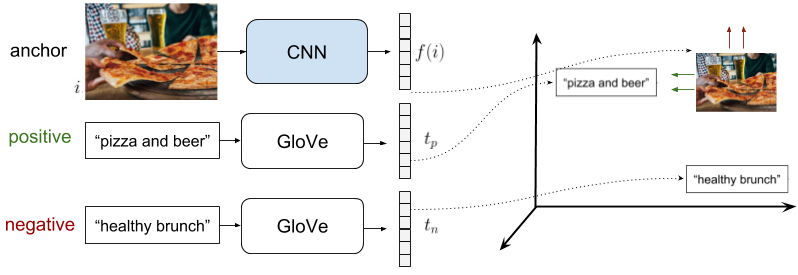Triplet Ranking Loss training of a multi-modal retrieval pipeline. The text GloVe embeddings are fixed, and we train the CNN to embed the image closer to its positive text than to the negative text.

In this setup we only train the image representation, namely the CNN. Being $$i$$ the image, $$f(i)$$ the CNN represenation, and $$t_p$$, $$t_n$$ the GloVe embeddings of the positive and the negative texts respectively, we can write:

Using this setup we computed some quantitative results to compare Triplet Ranking Loss training with Cross-Entropy Loss training. I’m not going to explain experiment details here, but the set up is the same as the one used in (paper, blogpost). Basically, we do some textual queries and evaluate the image by text retrieval performance when learning from Social Media data in a self-supervised way. Results using a Triplet Ranking Loss are significantly better than using a Cross-Entropy Loss.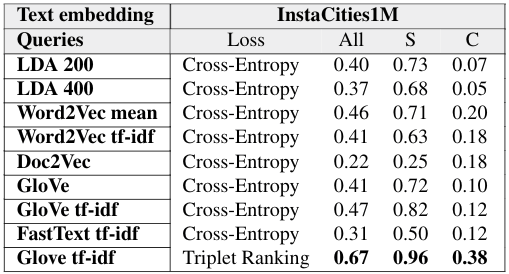Image retrieval by text average precision on InstaCities1M.

Another advantage of using a Triplet Ranking Loss instead a Cross-Entropy Loss or Mean Square Error Loss to predict text embeddings, is that we can put aside pre-computed and fixed text embeddings, which in the regression case we use as ground-truth for out models. That allows to use RNN, LSTM to process the text, which we can train together with the CNN, and which lead to better representations.

Similar approaches are used for training multi-modal retrieval systems and captioning systems in COCO, for instance in here.

## Ranking Loss Layers in Deep Learning Frameworks

### PyTorch

• CosineEmbeddingLoss. It’s a Pairwise Ranking Loss that uses cosine distance as the distance metric. Inputs are the features of the pair elements, the label indicating if it’s a positive or a negative pair, and the margin.

• MarginRankingLoss. Similar to the former, but uses euclidian distance.

• TripletMarginLoss. A Triplet Ranking Loss using euclidian distance.